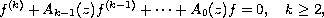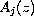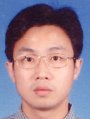Electron. J. Diff. Equ., Vol. 2010(2010), No. 81, pp. 1-11.

### Oscillation of higher-order linear differential equations with entire coefficients Zhi Gang Huang, Gui Rong Sun

Abstract:where coefficientsare entire functions of integer order. We obtain estimates on the orders and the hyper orders of the solutions to the above equation.Zhi Gang Huang School of Mathematics and Physics Suzhou University of Science and Technology Suzhou, 215009, China email: alexehuang@yahoo.com.cn Gui Rong Sun School of Mathematics and Physics Suzhou University of Science and Technology Suzhou, 215009, China email: sguirong@pub.sz.jsinfo.net